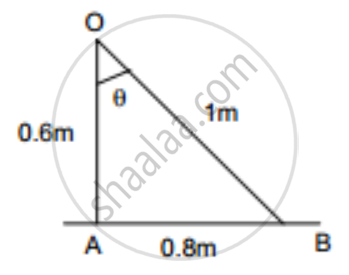Department of Pre-University Education, KarnatakaPUC Karnataka Science Class 12

# A Point Source Emitting Light Uniformly in All Directions is Placed 60 Cm Above a Table-top. the Illuminance at a Point on the Table-top, Directly Below the Source, is 15 Lux. - Physics

Sum

A point source emitting light uniformly in all directions is placed 60 cm above a table-top. The illuminance at a point on the table-top, directly below the source, is 15 lux. Find the illuminance at a point on the table-top 80 cm away from the first point.

#### Solution

Given,

Distance of the source from the table-top (r) = 60 cm or 0.6 m

Let Io be the intensity of illumination.

Illuminance directly below the source (EA) is given by,

E_A=I_0/(0.6)^2

I0 = 15 × (0.6)2
= 5.4 candelaLet EB be the illuminance at a point 80 cm away from the initial point.

So, E_B=(I_0 costheta)/(OB)^2

From the figure, we get

cos theta=0.6/1

OB = 1 m

Substituting the respective values in the above formula, we get

 E_B= 5.4(0.6/1)

= 3.24 lux

So, the illuminance at a point on the table-top 80 cm away from the first point is 3.24 lux.

Concept: Light Process and Photometry
Is there an error in this question or solution?

#### APPEARS IN

HC Verma Class 11, Class 12 Concepts of Physics Vol. 1
Chapter 22 Photometry
Exercise | Q 11 | Page 455
Share